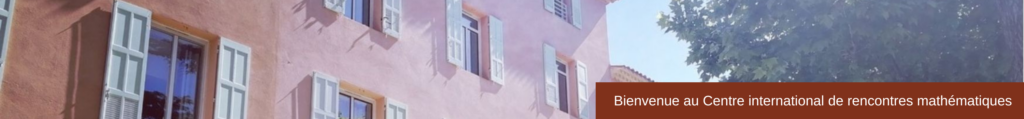OTHER EVENTS

Opening Day
September 2014
FRUMAM (CNRS, Université Aix-Marseille)

• 9h45: coffee, opening.
• 10h15: Hans-Georg FEICHTINGER (NuHaG, Department of Mathematics, University of Vienna, Austria): Function Spaces and Harmonic Analysis.
It is one of the facts of life that operators have certain mapping properties (e.g. the Fourier transform, the differentiation operator, certain convolution operators) and that it needs a repertoire of function spaces in order to properly describe the mapping properties of the different operators. New operators or classes of operators often initiate to introduction or great interest in new families of spaces. Interpolation theory provides good tools to view such spaces as families and conclude from mapping properties of operators « at the limiting cases of the parameter space’’ to the general situation (one may think of the Hausdorff Young theorem).
One of the goals of this presentation was a view on Besov spaces, viewed as generalized Lipschitz spaces, or as spaces characterized by dyadic decomposition of the Fourier domain, or finally as coorbit spaces with respect to the canonical representation of the ax+b-group on the Hilbert space L^2(R).
• 11h00: Hartmut FÜHR (Lehrstuhl A für Mathematik, RWTH Aachen, FRG): Continuous Wavelet Analysis in Higher Dimensions.
The continuous wavelet transform, introduced by Grossmann, Morlet and Paul for one-dimensional signals, generalizes to higher dimensions in many distinct ways. Initial higher-dimensional analogs were based on the similitude groups, more recent constructions use anisotropic alternatives such as the shearlet.
In the talk Führ reviewied the group-theoretic construction of these systems. The approximation-theoretic properties of the various wavelet systems can be studied in a very general unified manner using coorbit theory. For most of the interesting properties of the generalized wavelet transform, a proper understanding of the dual action underlying the wavelet system is crucial.
• 12h00: lunch break.
• 14h00: Karlheinz GRÖCHENIG (NuHaG, Department of Mathematics, University of Vienna, Austria): Bergman Spaces on the Unit Ball and Coorbit Theory.
Coorbit theory is a general machine to define Banach spaces and to derive atomic decompositions and Banach frames. Its starting point is a (square) integrable, irreducible unitary representation of a locally compact group.
For instance, the coorbit spaces associated to the group of affine translations are just the Besov space, and the corresponding atomic decompositions are their wavelet expansions. In this talk Gröchenig explained the coorbit spaces arising from the discrete series of SU(n,1) and their atomic decompositions. The coorbits attached to these representations were the classical Bergman spaces on the unit ball in \$C^n\$. The corresponding atomic decompositions were those derived by Coifman and Rochberg with complex-analytic methods.
(This is joint work with Jens G. Christensen and G. Olafsson).
• 14h45: Radu BALAN (Department of Mathematics and Center for Scientific Computation and Mathematical Modeling, University of Maryland, USA): Phase Retrieval using Lipschitz Continuous Maps.
In this talk Balan showed that reconstruction from magnitudes of frame coefficients (the so called « phase retrieval problem ») can be performed using Lipschitz continuous maps. Specifically we show that when the nonlinear analysis map a:H -> R^m is injective, with (a(x))_k = |<x,fk>|^2, where {f1, …, fm} is a frame for the Hilbert space H, then there exists a left inverse map w:R^m->H that is Lipschitz continuous.
Additionally we obtain the Lipschitz constant of this inverse map in terms of the lower Lipschitz constant of a.